International
Tables for
Crystallography
Volume B
Reciprocal space
Edited by U. Shmueli

International Tables for Crystallography (2010). Vol. B, ch. 2.5, pp. 348-349   | 1 | 2 |

## Section 2.5.3.4.2. Point-group determination

M. Tanakaf

#### 2.5.3.4.2. Point-group determination

| top | pdf |

The symmetries of the CBED patterns can be determined by examination of the symmetries of the structure factor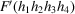in equations (2.5.3.7)or (2.5.3.8). We consider a displacive modulated structure, which has a modulation wavevector k = k3c* and belongs to (3 + 1)-dimensional space group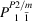. This space-group symbol implies the following.

 (1) The modulation wavevector k exists inside the first Brillouin zone of the average structure (P). (2) The average structure belongs to space group P2/m, the twofold rotation axis being parallel to the c axis. (3) The symmetry subsymbol 1, which is written beneath symmetry symbol 2, indicates that the modulation wavevector k is transformed into itself by symmetry operation 2 of the average structure. The symmetry subsymbol beneath symmetry symbol m indicates that the wavevector k is transformed into −k by symmetry operation m. The modulated structure has a twofold rotation axis, which is common to the average structure, but does not have mirror symmetry, which is possessed by the average structure.

For the twofold rotation axis (symbol 2) of this space group, the structure factoris written as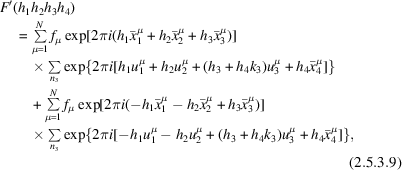where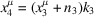. It is found from equation (2.5.3.9)that two structure factorsand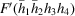are the same when reflections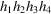and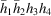are equivalent with respect to the twofold rotation axis of the average structure. Thus, not only fundamental reflections (h4 = 0) from the average structure but also the satellite reflections (h4 ≠ 0) from the incommensurate structure show twofold rotation symmetry about the c* axis.

For the mirror plane (symbol m), the structure factor is written in a similar manner to the case of the twofold rotation axis. It is found thatis not equal to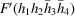for the incommensurate reflections h4 ≠ 0. Hence, the incommensurate reflections do not show mirror symmetry with respect to the mirror plane of the average structure. For the fundamental reflections (h4 = 0),is equal to, indicating the existence of mirror symmetry. It should be noted that the mirror symmetry can be destroyed by the dynamical diffraction effect between the fundamental and incommensurate reflections. In most modulated structures, however, the amplitude of the modulation wave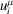is not so large as to destroy the symmetry of the fundamental reflections. Thus, the fundamental reflections ought to show the symmetry of the average structure, while the incommensurate reflections lose the symmetry.

The problem of the finite size of the illuminated area is discussed using equations (2.5.3.7)and (2.5.3.8)in a paper by Terauchi & Tanaka (1993) and in the book by Tanaka et al. (1994, pp. 156–205). The results are as follows: Even if the size and position of an illuminated specimen area are changed, the intensity distribution in a CBED pattern changes but the symmetry of the pattern does not. To obtain the symmetries of incommensurate crystals, it is not necessary to take CBED patterns from an area whose diameter is larger than the period of the modulated structure. The symmetries of the modulated structure can appear when more than one unit cell of the average structure is illuminated for displacive modulations. For substitutional modulations, a specimen volume that produces the average atom form factor is needed, namely a volume of about 1 nm diameter area and 50 nm thick.

Table 2.5.3.13shows the point-group symmetries (third column) of the incommensurate reflections for the two point-group subsymbols. For symmetry subsymbol 1, both the fundamental and incommensurate reflections show the symmetries of the average structure. For symmetry subsymbol, the fundamental reflections show the symmetries of the average structure but the incommensurate reflections do not have any symmetry. These facts imply that the symmetries of the incommensurate reflections are determined by the point group of the average structure and the modulation wavevector k. In other words, observation of the symmetries of the incommensurate reflections is not necessary for the determination of the point groups, although it can ascertain the point groups of the modulated crystals.

 Table 2.5.3.13| top | pdf | Wavevectors, point- and space-group symbols and CBED symmetries of one-dimensionally modulated crystals
Wavevector transformationPoint-group symbolSymmetry of incommensurate reflectionSpace-group symbolDynamical extinction lines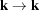1 Same symmetry as average structure 1, s (1/2), t (±1/3), q (±1/4), h (±1/6) Yes for s, q and h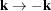No symmetryNo

An example of point-group determination is shown for the incommensurate phase of Sr2Nb2O7. Many materials of the A2B2O7 family undergo phase transformations from space group Cmcm to Cmc21 and further to P21 with decreasing temperature. An incommensurate phase appears between the Cmc21 phase and the P21 phase. Sr2Nb2O7 transforms at 488 K from the Cmc21 phase into the incommensurate phase with a modulation wavevector k = (½ − δ)a* (δ = 0.009–0.023) but does not transform into the P21 phase. The space group of Sr2Nb2O7 was reported as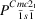(Yamamoto, 1988). (Since the space-group notation Cmc21 is broadly accepted, the direction of the modulation is taken as the a axis.) The point group of the phase is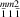. The modulation wavevector k is transformed to −k by the mirror symmetry operation perpendicular to the a axis () and by the twofold rotation symmetry operation about the c axis (). The wavevector is transformed into itself by the mirror symmetry operation perpendicular to the b axis ().

Fig. 2.5.3.19(a) shows a CBED pattern of the incommensurate phase of Sr2Nb2O7 taken with the  incidence at an accelerating voltage of 60 kV. The reflections indicated by arrowheads are the incommensurate reflections. Other reflections are the fundamental reflections. Since the pattern is produced by the interaction of the reflections in the zeroth-order Laue zone, symmetry operations () and () act the same. These symmetries are confirmed by the fact that the fundamental reflections show mirror symmetry perpendicular to the a* axis (twofold rotation symmetry about the c* axis) but the incommensurate reflections do not. Fig. 2.5.3.19(b) shows a CBED pattern of the incommensurate phase of Sr2Nb2O7 taken with the  incidence at 60 kV. The reflections in the two rows indicated by arrowheads are the incommensurate reflections and the others are the fundamental reflections. Symmetry symbol () implies that both the fundamental and incommensurate reflections display mirror symmetry perpendicular to the b* axis. Fig. 2.5.3.19(b) exactly exhibits the symmetry.Figure 2.5.3.19 | top | pdf |CBED patterns of the incommensurate phase of Sr2Nb2O7 taken at 60 kV. (a)  incidence: fundamental reflections show a mirror symmetry perpendicular to the a* axis but incommensurate reflections do not [symmetry ()]. (b)  incidence: incommensurate reflections show mirror symmetry perpendicular to the b* axis [symmetry ()]. The wave number vector of the modulation is k = (½ − δ)a*.

### References

Tanaka, M., Terauchi, M. & Tsuda, K. (1994). Convergent-Beam Electron Diffraction III. Tokyo: JEOL–Maruzen.
Terauchi, M. & Tanaka, M. (1993). Convergent-beam electron diffraction study of incommensurately modulated crystals. I. (3+1)-dimensional point groups. Acta Cryst. A49, 722–729.
Yamamoto, N. (1988). Electron microscope study of incommensurate phases. Kotaibutsui, 23, 547.